# Number Chart 1 -500 PrintableUpdated on Apr 19, 2022By Printablee Team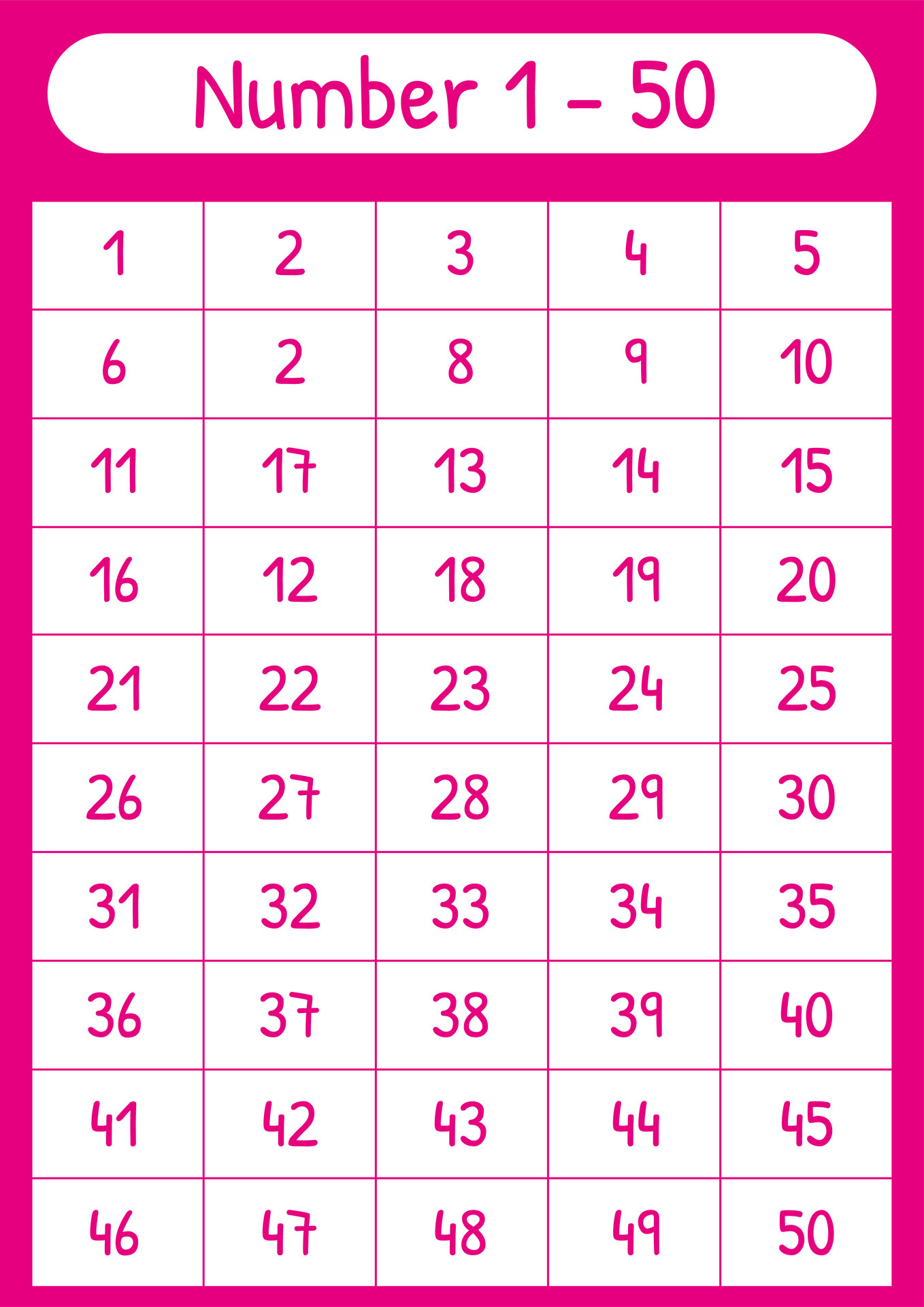## What are the Technique to Use for Counting for Kids?

Show the audience how to count forward and backward while introducing a number line. Children can better comprehend the idea of counting and the order of numbers thanks to this visual portrayal. To aid youngsters in remembering skip-counting patterns and the order of the numbers, use appealing songs or chants. The learning process may be made more interesting and remembered by repetition and rhythm.

As they count, encourage them to point to each number on the chart. We recommend number chat 100 because the number chart presents numbers 1-100 which are important for children to learn. It is a tactile learning experience since they can move or hop to each number physically. To draw attention to skip-counting patterns, use various colors.

Use a different color for multiples of 2, 3, and so on, for instance. Children may notice patterns and comprehend skip counting with the aid of this visual distinction.

Use manipulatives to represent the numbers on the chart, such as counters, blocks, or tiny items. Children can better see and comprehend the notion by grouping these items into groups based on skip-counting patterns. Children are asked to finish incomplete 1-100 number charts by adding the omitted digits. This activity helps kids learn to skip counting while reinforcing the counting pattern.

Integrate interactive counting and skip-counting games and activities in your curriculum. Apply real-world scenarios to counting and skip-counting. Children can better learn the practical implications of these abilities by having them count items in a grocery shop or skip numerals while counting steps, for example.

Introduce skip-counting techniques progressively after beginning with simple counting. To reaffirm comprehension and encourage retention, go through previously taught material frequently. Allow kids plenty of opportunities to practice counting and skip-counting with number charts. Their knowledge and numerical fluency are strengthened with regular practice.

## What is Counting Chart?

Visual representations of numbers placed in a grid or order are called number charts or counting charts. Children and learners can use them to improve their number identification, counting abilities, and comprehension of number sequences. In a counting chart, the numbers are often shown in a row or column arrangement, frequently beginning at zero or one and going up to a particular number.

By changing the activities and level of difficulty of the concepts being introduced, the Counting Chart can be efficiently changed and used at different grade levels. Here are a few modifications for various grade levels:

• Early Elementary
• Upper elementary
• Middle school
• High school

## How Do the Number Counting Charts Use for Learning?

Depending on the individual aims and age range of the learners, counting charts may be utilized in a variety of ways. Here are a few examples:

• Number Recognition: Counting charts assist kids in learning how to identify and recognize numbers. They may use the chart to point to each number, which will help them connect the number to the matching amount.

• Counting Practice: Learners can practice counting aloud or in a whisper by using counting charts. They can count up or down from a specific starting point, reinforcing their counting abilities and increasing number fluency.

• Skip-Counting: Counting by a particular interval or pattern, such as by twos, fives, or tens, is known as skip counting. Counting charts are particularly helpful in this situation. The chart's visual design makes it easier for students to recognize the skip-counting pattern and comprehend the idea.

• Number Patterns: Students can spot number patterns like multiples of a particular number or even, odd, or even numbers by looking at the counting chart. This enhances their capacity for pattern recognition.

• Basic Arithmetic: Counting charts can also be used to introduce fundamental addition and subtraction concepts in basic arithmetic. Students can add or subtract a specific amount while keeping track of the numbers visually.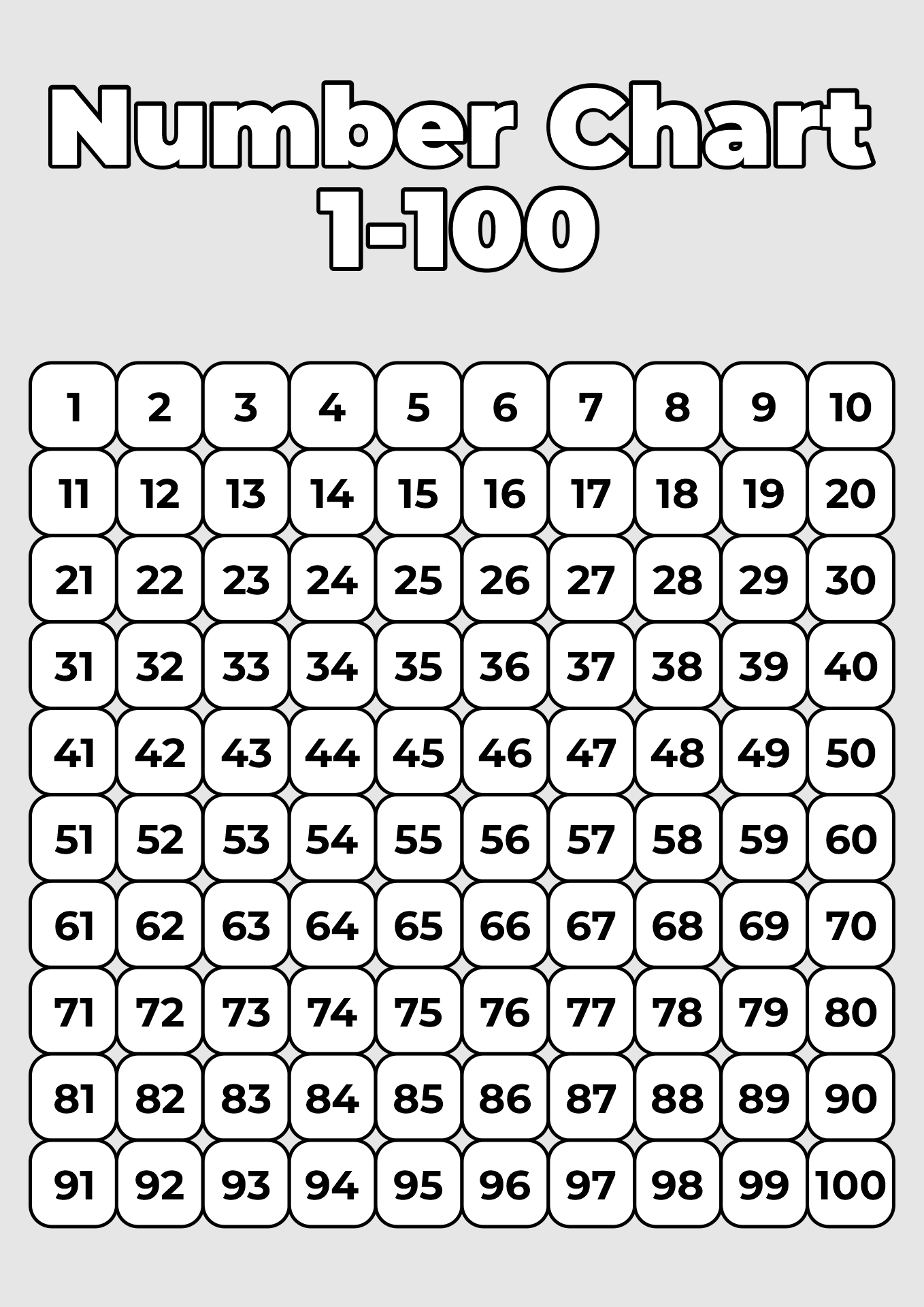We also have more printable chart you may like:
Printable Multiplication Chart 1-20
Numbers From 1-100 Chart Printable
Printable Numbers 100 Chart 1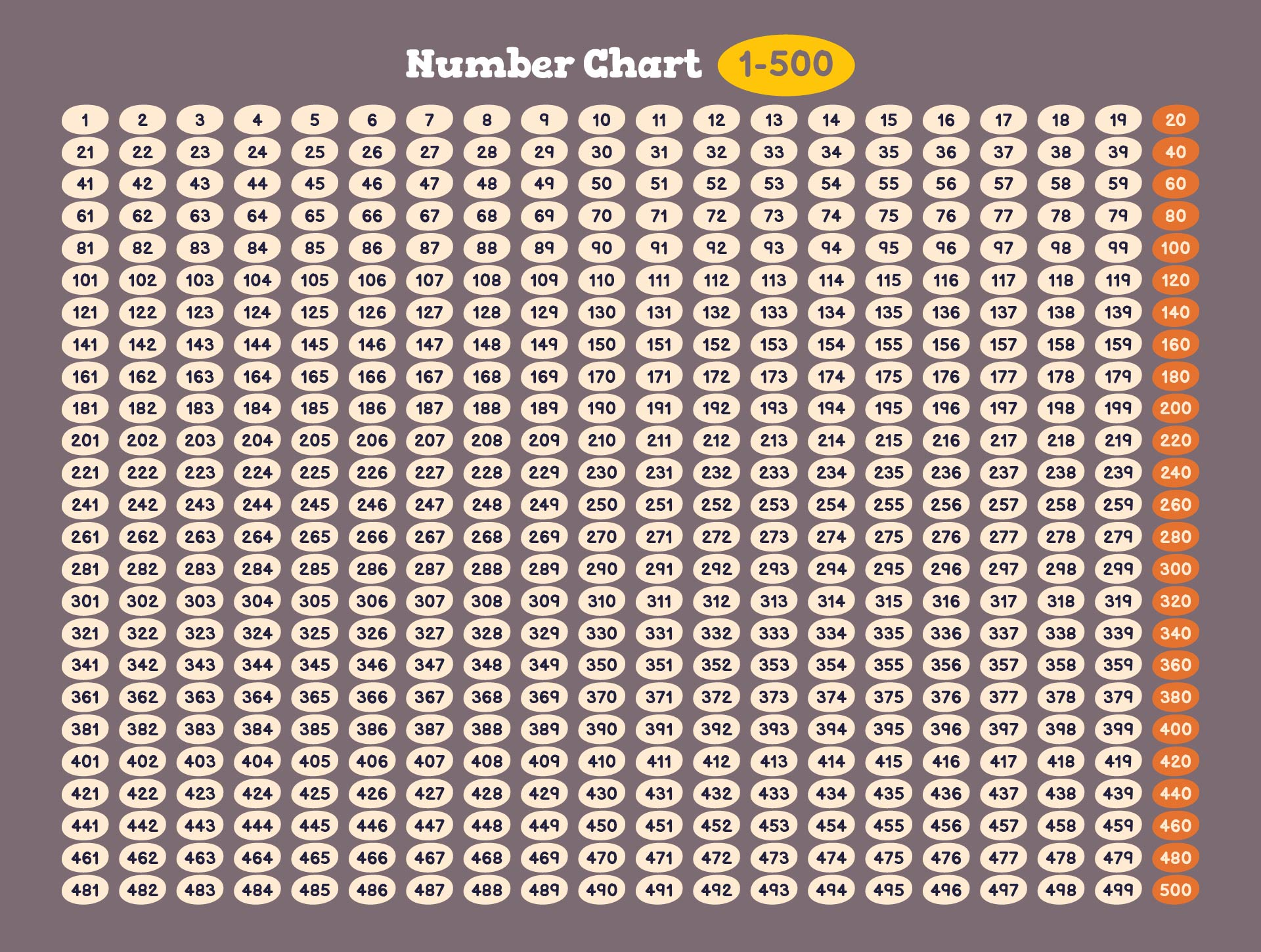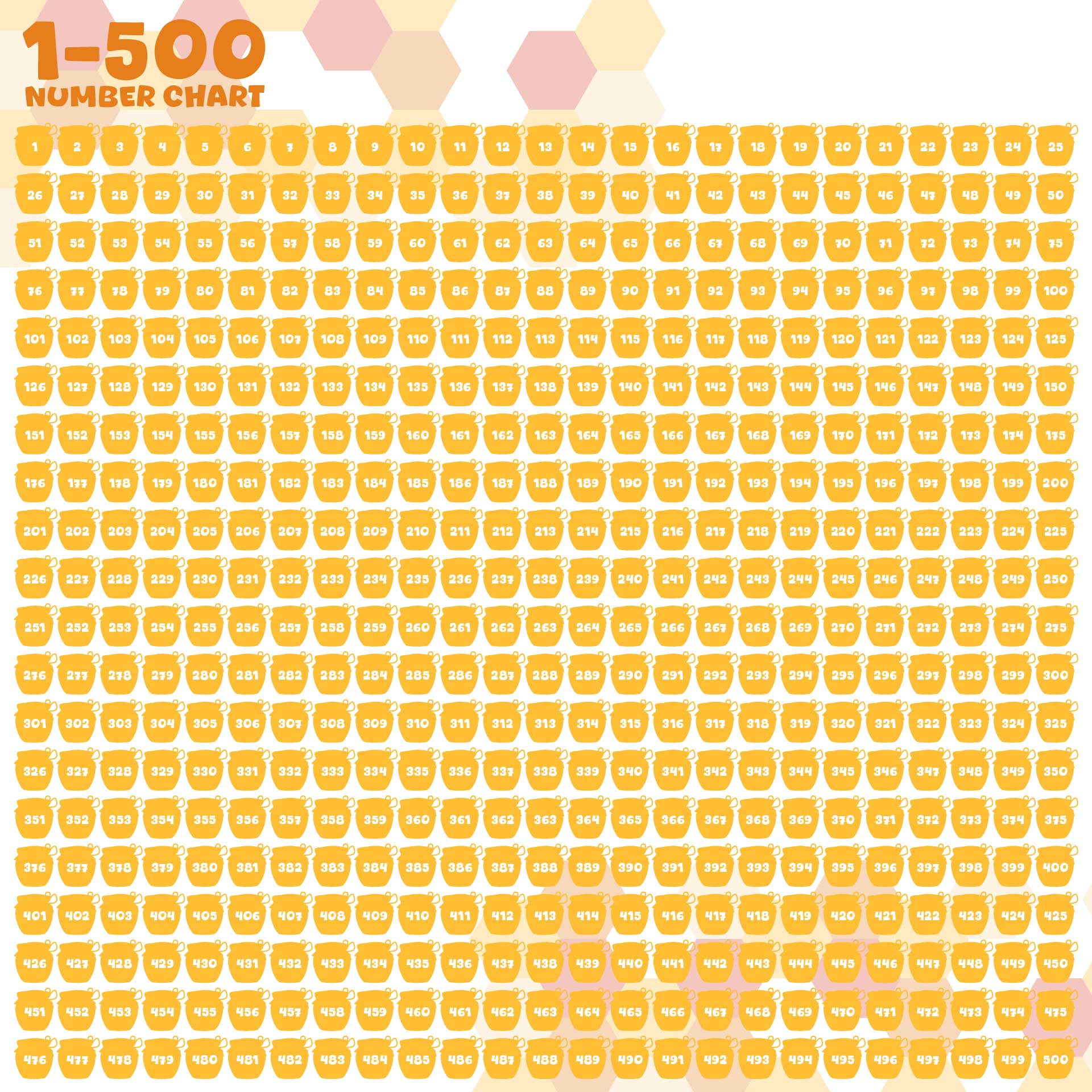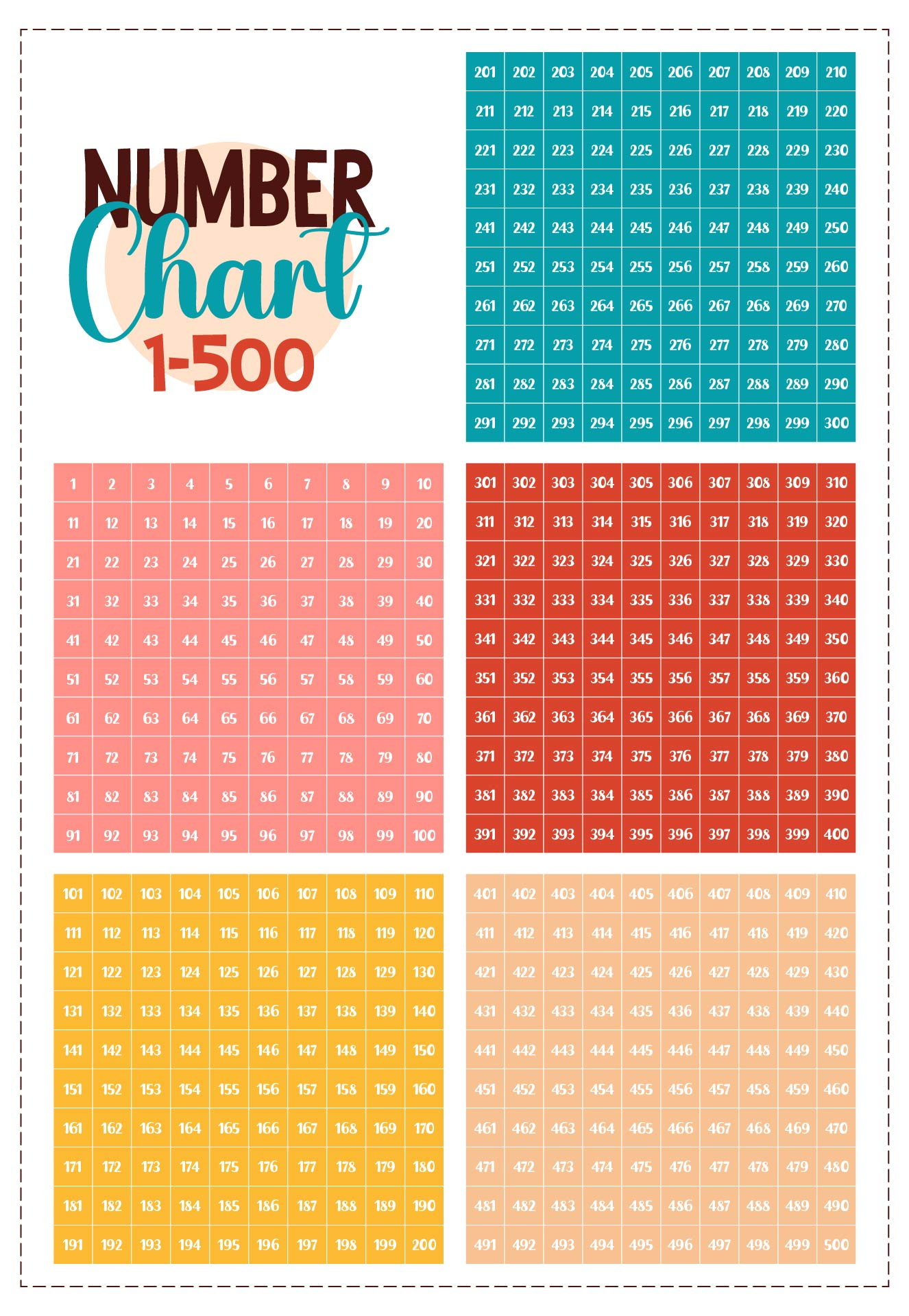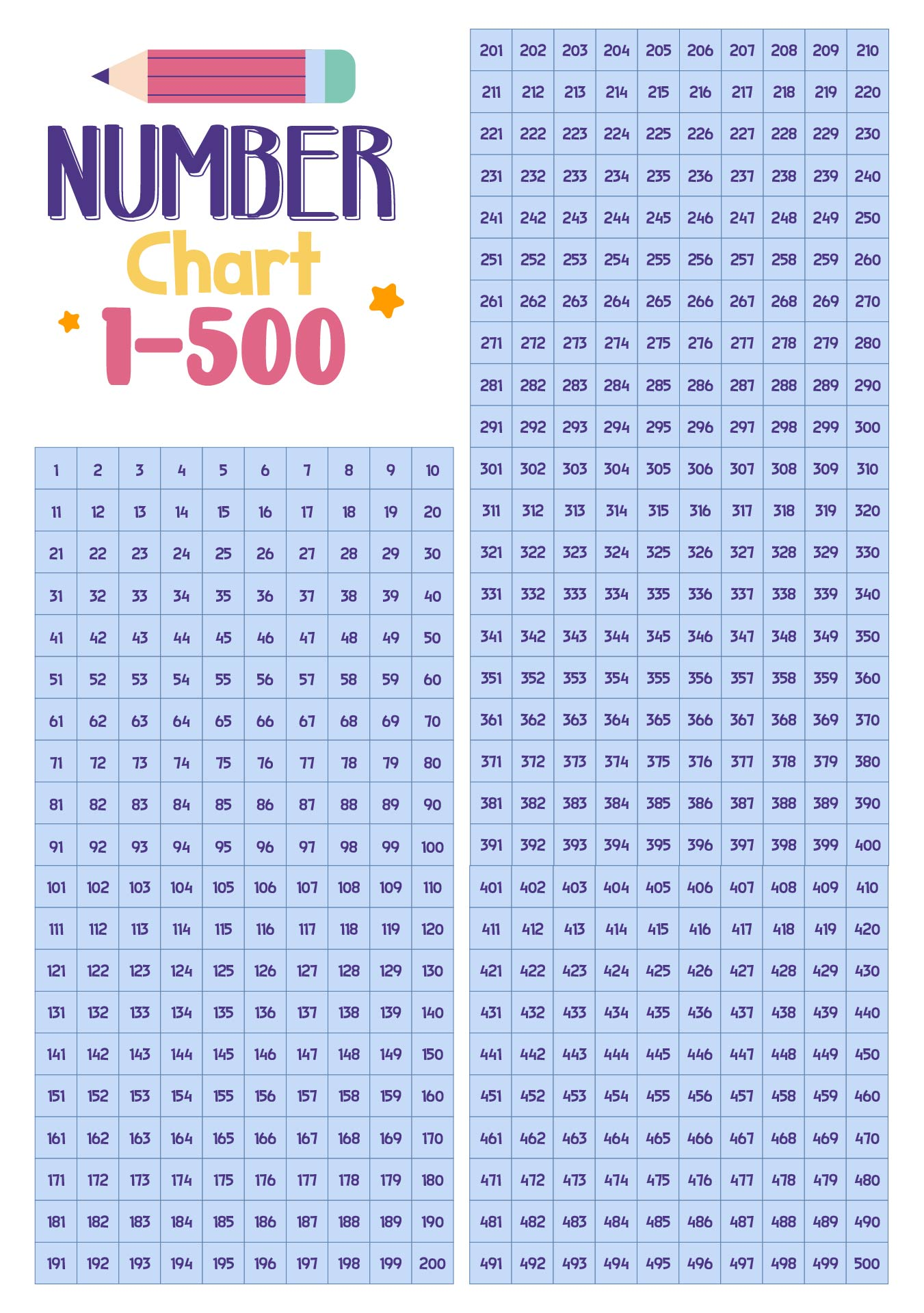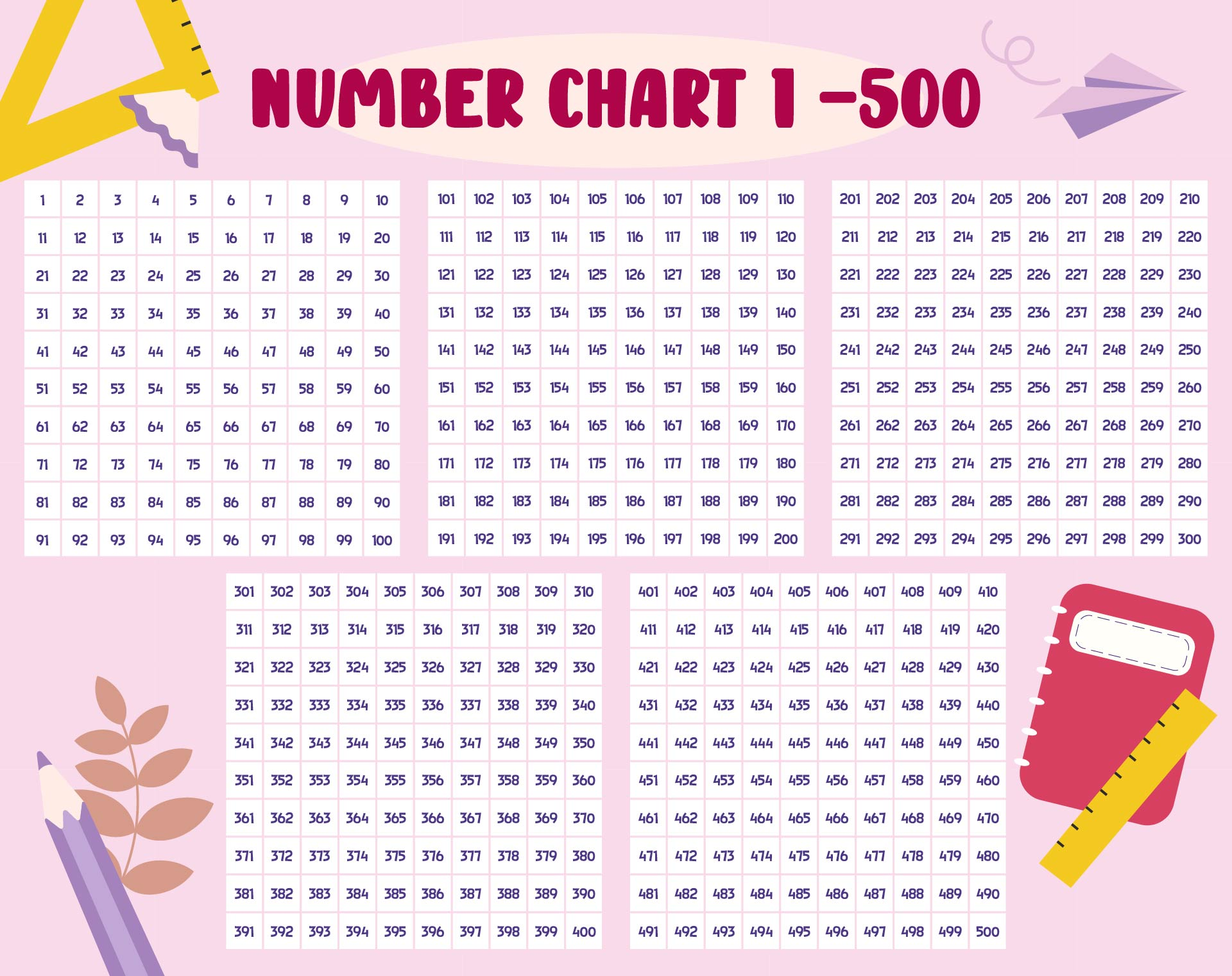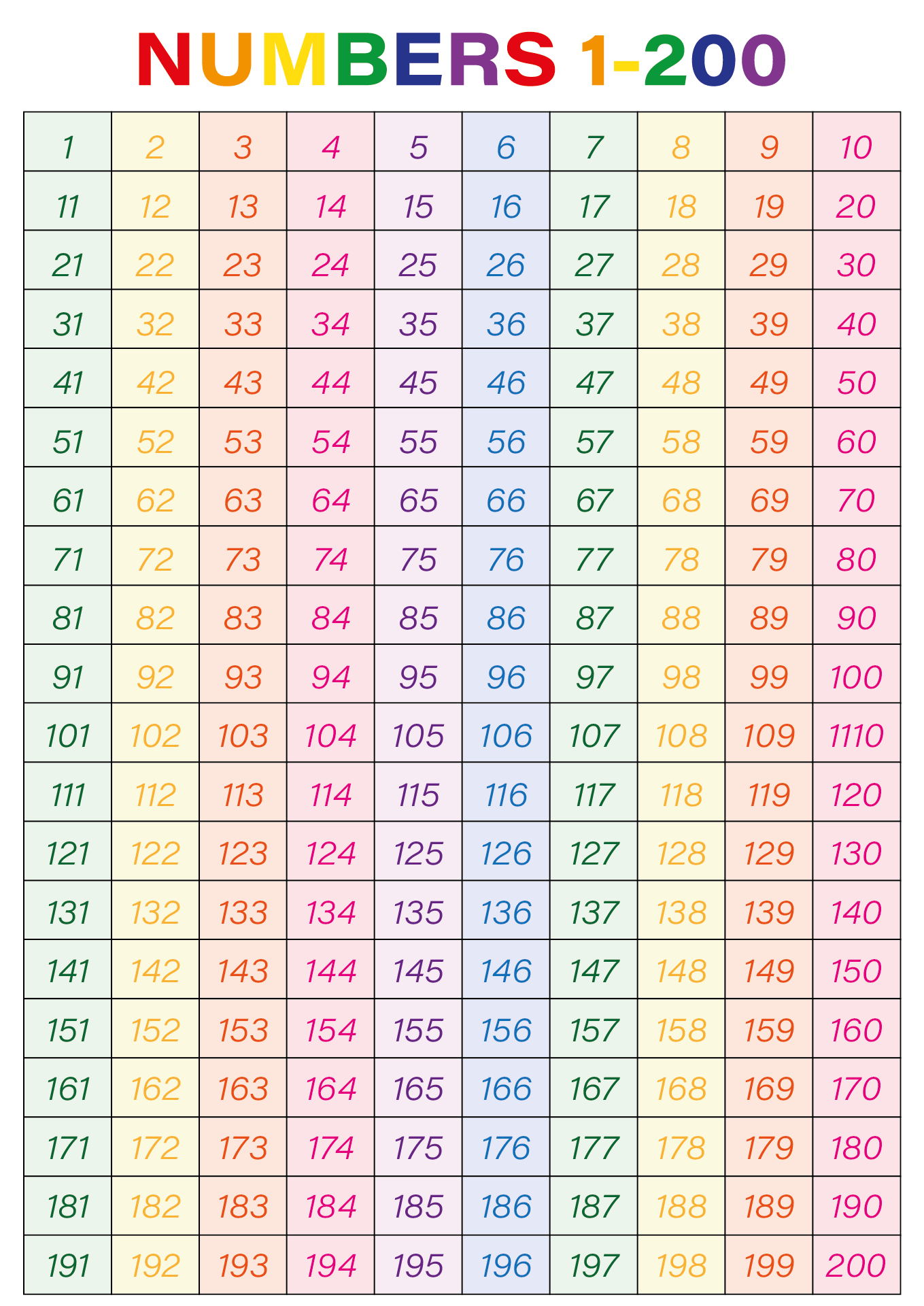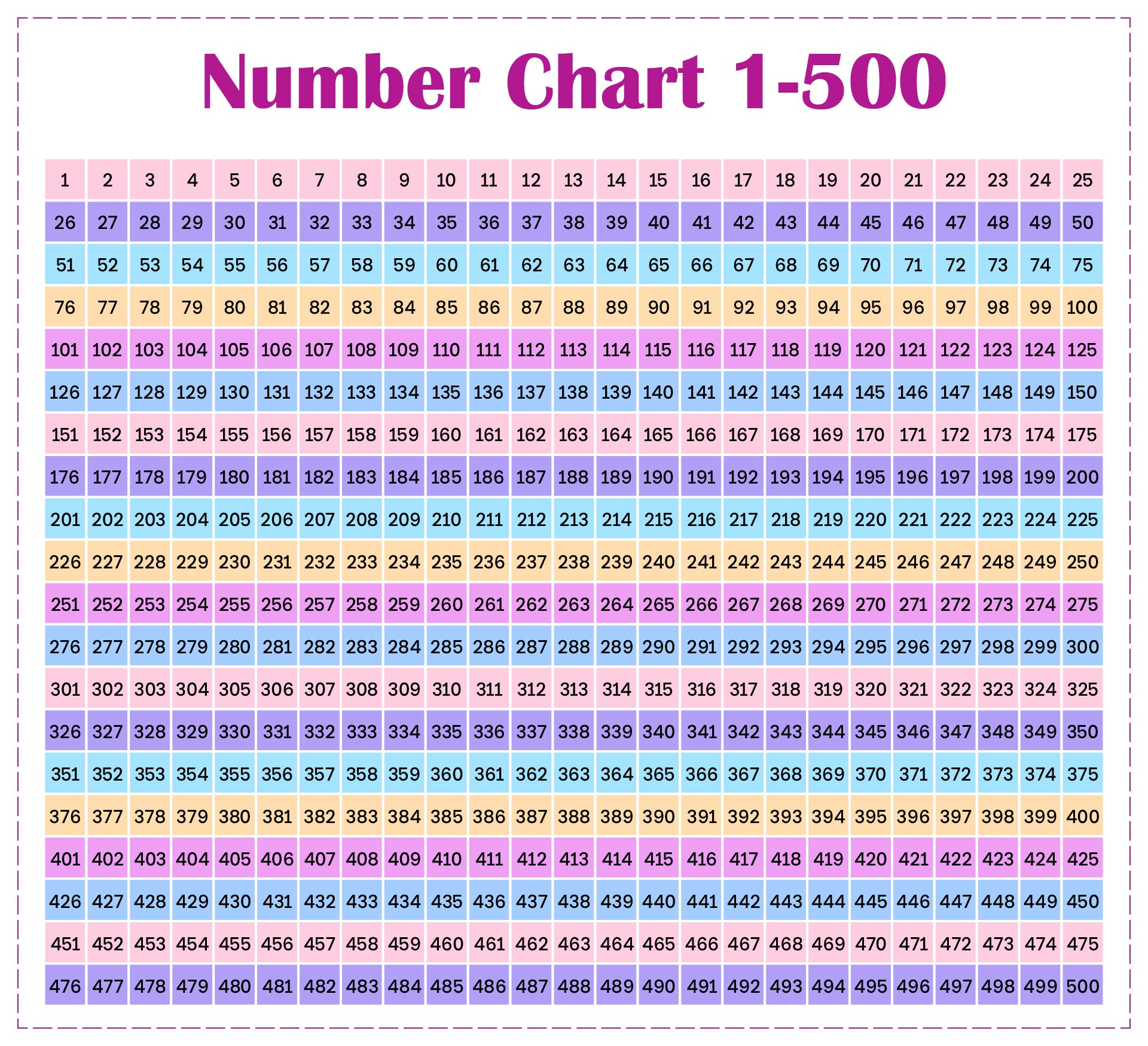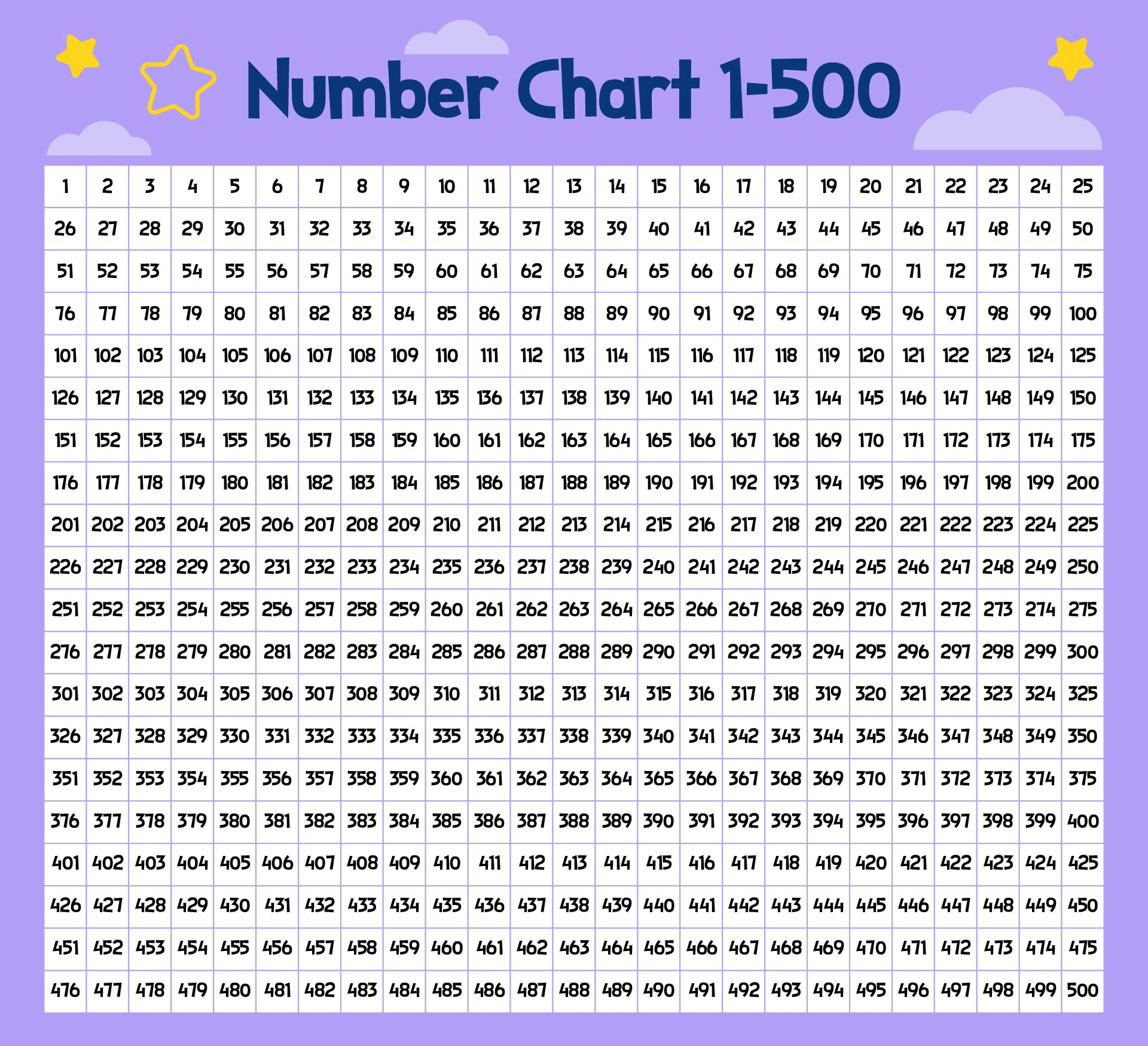## How Can the Counting Chart be Used to Teach Multiplication and Division?

While the counting chart is a helpful tool, it is vital to combine it with additional teaching techniques and practical exercises to guarantee that students have a thorough comprehension of multiplication and division ideas.

One of the best techniques for teaching numbers to children is to use paint by number activities. Children have to color certain areas of the picture. There are numbers in each area. If children understand the concept of numbers, you can easily teach counting charts through multiplication and division.

Multiplication: Use the counting chart to illustrate multiples of a particular number graphically. This aids in their pattern recognition and comprehension of the multiplication idea.

Arrays and groups can be represented using a counting chart. Understanding multiplication as repeated addition is made easier by this graphic portrayal. The chart may be used to learn skip counting, which is a kind of multiplication.

Division: Learners can use the counting chart to visually illustrate equal sharing when introducing division. This makes it easier to comprehend division as the division of things into equal groups. Understanding the connection between division and multiplication is another benefit of using the counting chart.

Learners can recognize fact families by recognizing number patterns and the multiplication and division facts that go along with them. The chart can help in determining a number's factors. Learners can determine the factors by locating all the numbers that divide into a given number equally.

Problem-solving: Counting charts can be used as visual assistance while creating word problems that involve multiplication or division. The chart can be used by students to understand how division works and arrive at a solution.

You can use the counting chart as a guide when learning your multiplication facts. As they practice multiplication, students may see how certain numbers relate to one another.